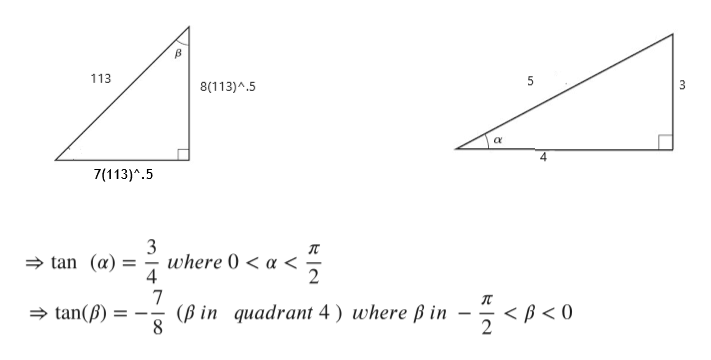Hello, find the exact value of each of the following under the given conditions. sin alpha=3/5, 0<alpha<pi/2  ;   cos beta= (8sqrt113)/113, -pi/2<beta<0 (a) sin (alpha+beta)= -(4sqrt113)/565(b) cos (alpha+beta)= (53sqrt113)/565(c) sin (alpha-beta)= (52sqrt113)/565(d) tan (alpha-beta)= ? I got the answers for (a to c), the correct answers, but I couldn't get (d) correct. I got the answer 53/4 instead of 52/11, which is the correct answer for (d). I used the sum and difference formulas for each of them, yet I don't understand how 52/11 is the correct answer. Can you explain how it is 52/11?

Question

Hello,

find the exact value of each of the following under the given conditions.

sin alpha=3/5, 0<alpha<pi/2  ;   cos beta= (8sqrt113)/113, -pi/2<beta<0

(a) sin (alpha+beta)= -(4sqrt113)/565

(b) cos (alpha+beta)= (53sqrt113)/565

(c) sin (alpha-beta)= (52sqrt113)/565

(d) tan (alpha-beta)= ?

I got the answers for (a to c), the correct answers, but I couldn't get (d) correct. I got the answer 53/4 instead of 52/11, which is the correct answer for (d). I used the sum and difference formulas for each of them, yet I don't understand how 52/11 is the correct answer. Can you explain how it is 52/11?

Step 1

We have to find value of tan(alpha-beta).

Information is given below:

Step 2

We will use following formula.

Formula is given below:

Step 3

Now use pythagorean theorem to find remaining sides of right angle triangles with angle alpha and beta.

alpha in ...help_outlineImage Transcriptionclose113 5 3 8(113)^.5 4 7(113)^.5 3 where 0 < a< tan (a) 4 7 (Bin quadrant 4) where ß in 2 tan() 8 11 fullscreen

Want to see the full answer?

See Solution

Want to see this answer and more?

Our solutions are written by experts, many with advanced degrees, and available 24/7

See Solution
Tagged in

Functions